cancel
Showing results for
Did you mean:Helper I

## How to exclude an entry from visualisation table if both fields are showing zeros

 Raw data Country Project ID Sub-project ID Chart of accounts Project Manager ID Variable Cost Fixed Cost Japan 779966 335566 93416 33 2,000 5,000 Japan 779966 335566 88334 33 1,000 - Japan 779966 335566 88334 33 - - Japan 779966 774455 93416 55 - - South Korea 663322 115577 93416 33 - 3,000 Above example is raw data. In visual table (below), I do not want to show the line if both Variable Cost AND Fixed Cost are zeros. How do I do that? Expected Table Visualisation Country Project ID Sub-project ID Chart of accounts Project Manager ID Variable Cost Fixed Cost Japan 779966 335566 93416 33 2,000 5,000 Japan 779966 335566 88334 33 1,000 - South Korea 663322 115577 93416 33 - 3,000

1 ACCEPTED SOLUTIONSuper User

Thanks for the sample data. It makes things much easier.

Seeing the dataset, the data contains rows which, when grouped by the IDs, sum out to 0, This means that filter expressions referenced to row value <> 0 wil still return these rows (they have values but their aggregated sum is 0). Here is an example: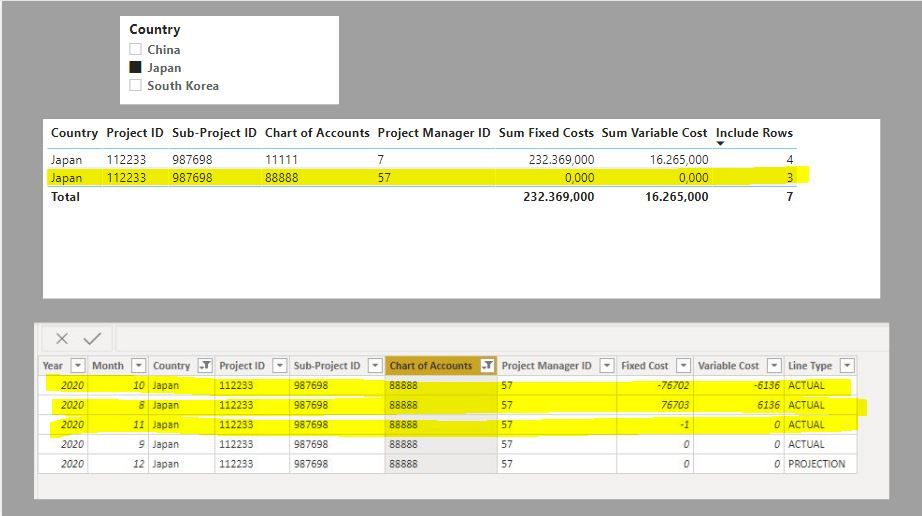If you want to exclude these rows since they sum to 0, use the following measure instead to filter the visual in the filter pane (set the value to "is greater or equal to 1")

``````Exclude Sum to 0 rows =
VAR Summ =
//This creates a virtual table summarizing the measures by the IDs/fields included
SUMMARIZE (
Table1,
Table1[Project ID],
Table1[Sub-Project ID],
Table1[Chart of Accounts],
Table1[Project Manager ID],
Table1[Line Type],
"Fixed", [Sum Fixed Costs],
"Variable", [Sum Variable Cost]
)
RETURN
//The measure filters the virtual table to count rows containing "ACTUAL" and with summarized values which are not 0
COUNTROWS (
FILTER (
Summ,
Table1[Line Type] = "ACTUAL"
&& OR ( [Fixed] <> 0, [Variable] <> 0 )
)
)``````

and you will get this result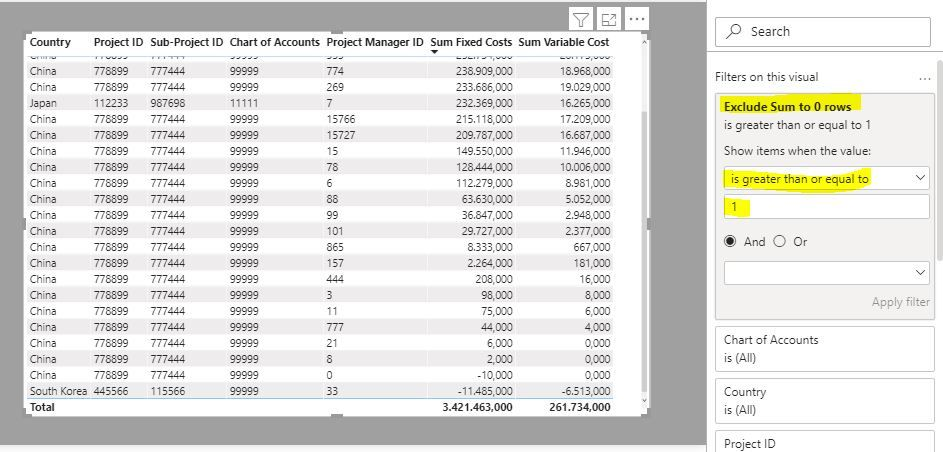In doing so, you are also helping me. Thank you!

Proud to be a Super User!

12 REPLIES 12Super User

Here is one way of doing this.

Create a measure to identify each row by a "fullID", filter the rows to only include rows with either values, and then use this measure in the "filters for this visual" in the filter pane (where the measure returns 1)

``````Include Rows =
VAR FullID = VALUES('DataTable'[Project ID]) & VALUES('DataTable'[Sub-project ID]) & VALUES('DataTable'[Chart of accounts]) & VALUES('DataTable'[Project Manager ID])
RETURN
COUNTROWS(
CALCULATETABLE(
FILTER('DataTable',
OR([Fixed Cost]>0,[Variable Cost] >0))))``````

To get this:In doing so, you are also helping me. Thank you!

Proud to be a Super User!Helper I

Hi @PaulDBrown I tried your suggested method. It doesn't quite work on my real dataset. I'm not quite sure if it is because my previous dataset example was too simplified. I am attaching the dataset in Excel below. These are the dataset where some lines work and some doesn't based on your suggested method.

P/S I only want to show "ACTUAL" (as per Pivot).Super User

Thanks for the sample data. It makes things much easier.

Seeing the dataset, the data contains rows which, when grouped by the IDs, sum out to 0, This means that filter expressions referenced to row value <> 0 wil still return these rows (they have values but their aggregated sum is 0). Here is an example:If you want to exclude these rows since they sum to 0, use the following measure instead to filter the visual in the filter pane (set the value to "is greater or equal to 1")

``````Exclude Sum to 0 rows =
VAR Summ =
//This creates a virtual table summarizing the measures by the IDs/fields included
SUMMARIZE (
Table1,
Table1[Project ID],
Table1[Sub-Project ID],
Table1[Chart of Accounts],
Table1[Project Manager ID],
Table1[Line Type],
"Fixed", [Sum Fixed Costs],
"Variable", [Sum Variable Cost]
)
RETURN
//The measure filters the virtual table to count rows containing "ACTUAL" and with summarized values which are not 0
COUNTROWS (
FILTER (
Summ,
Table1[Line Type] = "ACTUAL"
&& OR ( [Fixed] <> 0, [Variable] <> 0 )
)
)``````

and you will get this resultIn doing so, you are also helping me. Thank you!

Proud to be a Super User!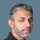Super User

@gracechong

If both Variable and Fixed cost are zero then, the grand totals for these fields will stay the same regards of the filter.

Are you referring to any other columns other than these where you have grand totals?

________________________

If my answer was helpful, please consider Accept it as the solution to help the other members find it

Click on the Thumbs-Up icon if you like this reply 🙂

Did I answer your question? Mark my post as a solution! and hit thumbs upHelper I

hi @Fowmy

the grand totals that i'm referring to are the grand totals of the 2 fields in visualisation table. so meaning, if originally before any changes, the variable cost grand total should be 3000 while fixed cost grand total should be 8000 based on above example. this is a control check as to whether the suggestion works. but when i use the filter pane "variable cost is not zero" and "fixed cost is not zero", the grand total changed to a lesser amount. so it is indicating that this suggestion eliminated some lines that should not have been eliminated.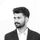Community Champion

Write a DAX measure and apply that in the visual level filter.

``````ShowHide =
VAR _variableCost = [Variable Cost]
VAR _fixedCost = [Fixed Cost]
VAR _result =
IF ( AND ( _variableCost = 0, _fixedCost = 0 ), "hide", "show" )
RETURN
_result``````

set the filter condition as ShowHide is Show.

Regards,
Nandu Krishna

Appreciate with a kudos 👍

Proud to be a Super User!Helper I

Hi @nandukrishnavs this solution is close. Good thing is grand total remains the same. However while eliminating some lines where both are zeros, it is however still showing certain lines where both values are zeros. Not sure what could be the cause?Community Champion

Updated the measure logic.

``````ShowHide =
VAR _variableCost = [Variable Cost]
VAR _fixedCost = [Fixed Cost]
VAR _result =
IF (
AND (
_variableCost = 0
|| ISBLANK ( _variableCost ),
_fixedCost = 0
|| ISBLANK ( _fixedCost )
),
"hide",
"show"
)
RETURN
_result``````

Regards,
Nandu Krishna

Appreciate with a kudos 👍

Proud to be a Super User!Helper I

Hi, the updated measure above doesn't work. I am attaching the raw data here in case you are able to figure out what field is causing the measure to not work.

P/S I only want to show "ACTUAL" (as per Pivot).Community Champion

Based on your raw data, I have created a calculated column.

``ShowHideColumn = IF(AND('Table'[Fixed Cost]=0,'Table'[Variable Cost]=0),"Hide","Show")``

Then applied it in the visual level filter. Please refer to the attached pbix file.Super User

@gracechong

you can use the Filter Pane to set both Variable and Fixed Cost filter to NOT EQUAL BLANK one by one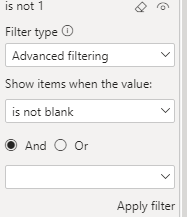________________________

If my answer was helpful, please consider Accept it as the solution to help the other members find it

Click on the Thumbs-Up icon if you like this reply 🙂

Did I answer your question? Mark my post as a solution! and hit thumbs upHelper I

Hi @Fowmy , this method doesn't work as the entries are actually not null/ blank values. They are actual zeros. I also tried to apply these in filter pane if they are not zeros for both "Variable Cost" and "Fixed Cost" fields but it doesn't work in the sense that it changes the final grand total amount. By right if it works, it shouldn't change grand total amount as we are only excluding lines that are zero for both.